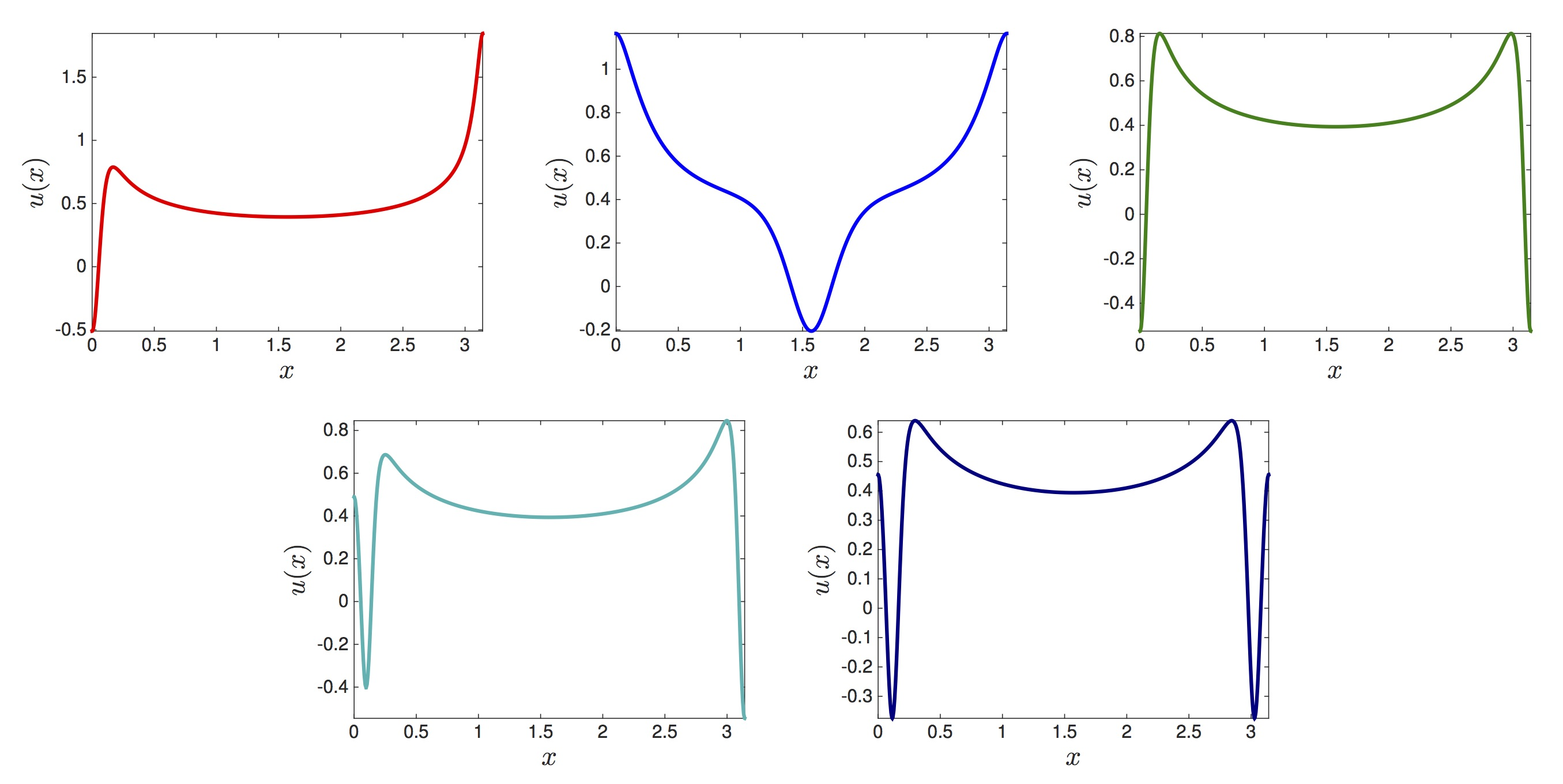### Computer assisted Fourier analysis in sequence spaces of varying regularity

Jean-Philippe Lessard and Jason D. Mireles James

This work treats a functional analytic framework for computer assisted Fourier analysis  which can be used to obtain mathematically rigorous error bounds on numerical approximations of solutions of differential equations. An abstract a-posteriori theorem is employed in order to obtain existence and regularity results for $C^k$ problems with $0 < k= \infty$ or $k= \omega$. The main tools are certain infinite sequence spaces of rapidly decaying coefficients: we employ sequence spaces of algebraic and exponential decay rates in order to characterize the regularity our results. We illustrate the implementation and effectiveness of the method in a variety of regularity classes. We also examine the effectiveness of spaces of algebraic decays for studying solutions of problems near the breakdown of analyticity.

The paper in its pdf form can be found here.

Here are the MATLAB codes to perform the computer-assisted proofs. They require installing and starting the interval arithmetic package INTLAB.
• Here, find the codes for the proofs in the $C^k$ category and in the analytic category.